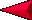Back SCIENTIFIC METHOD Lesson 3 - Page 1 NextLESSON 3.  Indirect Methods to Solve Problems (Lab)An equation is a mathematical statement in symbols that states how things are equivalent or the same.   Equations need to incorporate an equal sign. For example:  2+10 = 12.  Equations can help calculate unknown quantities if only certain properties are known.     Developing formulas that take into account properties can help figure out unknowns easier.   Equations follow rules and have defined properties in order to solve equations.   We will use volume, mass, and density to try and directly measure a quantity and then use a formula to find a quantity that you cannot measure.In ancient Greece there was a problem in how to measure volume of irregular objects.  Archimedes was the first to discover the Law of Buoyancy (Archimedes principle) which addresses this problem.  This law states that the mass of the displaced fluid is directly proportional to the volume of the displaced fluid. Rumor has it, that Archimedess was taking a bath and when he filled the water too high, it made him realize how fluid is displace.  He supposedly ran out of the tub into the city of Syracuse (Greece), naked, yelling Eureka, I found it.